﻿• 价格透明
• 信息保密
• 进度掌控
• 售后无忧# Transoformer再下一城之VSR-Transformer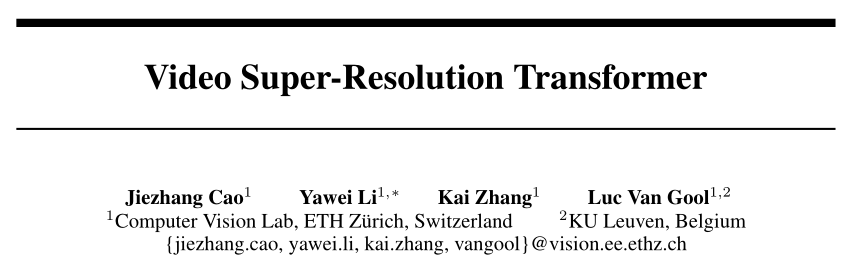# 看点

Transformer应用在VSR中有两大问题：

1. 全连接的自注意层由于依赖线性层来计算注意图而忽略了对局部信息的利用。
2. 由于词前馈层独立的处理每个输入，导致其缺乏特征对齐的能力。

# 方法

### overview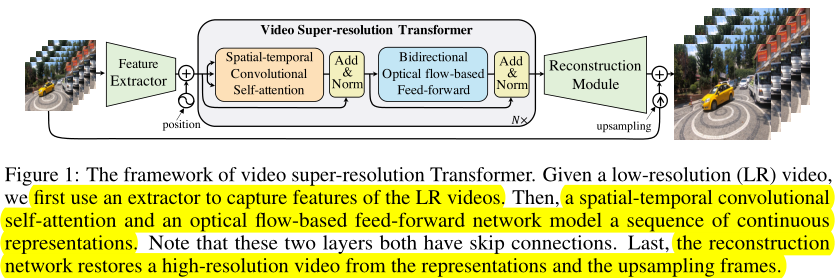### 时空卷积自注意层

Q = k 1 ( W q ∗ X ) ， K = k 1 ( W k ∗ X ) ， V = k 1 ( W v ∗ X ) Q=k_1(W_q * X)，K=k_1(W_k * X)，V=k_1(W_v * X) 其中， k 1 ( ⋅ ) k_1(·) 是展开操作，然后计算相似度矩阵 σ 1 ( Q T K ) σ_1(Q^TK) 并与值V聚合以获得特征图。最后，采用折叠操作 k 2 ( ⋅ ) k_2(·) 将这些滑动局部patches组合起来，并使用输出层 W o W_o 来输出最终特征图，公式如下：
f 1 ( X ) = ϕ ( X + ∑ i = 1 h W o i ∗ κ 2 ( κ 1 ( W v i ∗ X ) ⏟ V σ 1 ( κ 1 τ ( W k i ∗ X ) ⏟ K ⊤ κ 1 τ ( W q i ∗ X ) ⏟ Q ) ) ) f_{1}(\mathcal{X})=\phi\left(\mathcal{X}+\sum_{i=1}^{h} \mathcal{W}_{o}^{i} * \kappa_{2}(\underbrace{\kappa_{1}\left(\mathcal{W}_{v}^{i} * \mathcal{X}\right)}_{\mathcal{V}} \sigma_{1}(\underbrace{\kappa_{1}^{\tau}\left(\mathcal{W}_{k}^{i} * \mathcal{X}\right)}_{\mathcal{K}}^{\top} \underbrace{\kappa_{1}^{\tau}\left(\mathcal{W}_{q}^{i} * \mathcal{X}\right)}_{\mathcal{Q}}))\right) 其中， k 1 T ( ⋅ ) = τ ◦ k 1 ( ⋅ ) k_1^T(·)=τ◦k_1(·) 是reshape操作τ和展开操作 k − 1 k-1 的组合。本文中使用单头（h=1）获得了良好的性能。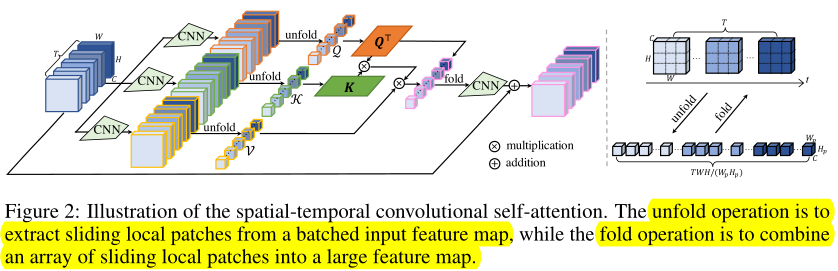P E (  pos  , i ) = { sin ⁡ ( pos ⁡ ⋅ α k ) ,  for  i = 2 k cos ⁡ ( pos ⁡ ⋅ α k ) ,  for  i = 2 k + 1 \mathrm{PE}(\text { pos }, i)=\left\{\begin{array}{ll} \sin \left(\operatorname{pos} \cdot \alpha_{k}\right), & \text { for } i=2 k \\ \cos \left(\operatorname{pos} \cdot \alpha_{k}\right), & \text { for } i=2 k+1 \end{array}\right. 其中， α k = 1 / 1000 0 2 k / d 3 \alpha_{k}=1 / 10000^{2 k / \frac{d}{3}} ，k是[0,d/6)中的一个整数，‘pos’表示维度位置，d是通道维度。

### 双向光流前馈层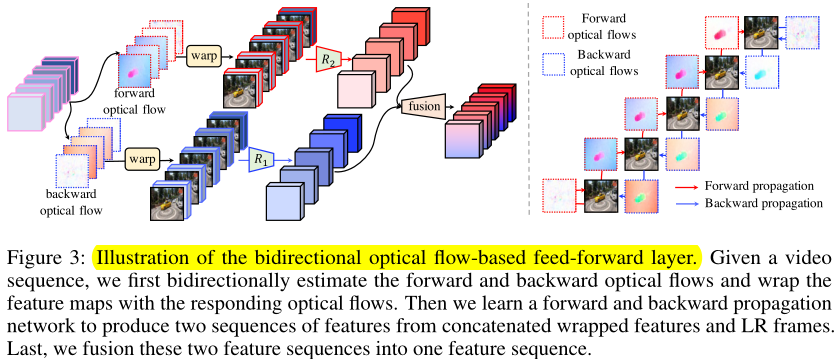f 2 ( X ) = ϕ ( f 1 ( X ) + ρ ( W ˇ 1 ∗ σ 2 ( W ˇ 2 ∗ [ V , X ‾ ] ) ⏟ backward propagation  + W → 1 ∗ σ 2 ( W → 2 ∗ [ V , X → ] ) ⏟ forward propagation  ) ) f_{2}(\mathcal{X})=\phi\left(f_{1}(\mathcal{X})+\rho(\underbrace{\check{\boldsymbol{W}}_{1} * \sigma_{2}\left(\check{\boldsymbol{W}}_{2} *[\mathcal{V}, \overline{\mathcal{X}}]\right)}_{\text {backward propagation }}+\underbrace{\overrightarrow{\boldsymbol{W}}_{1} * \sigma_{2}\left(\overrightarrow{\boldsymbol{W}}_{2} *[\mathcal{V}, \overrightarrow{\mathcal{X}}]\right)}_{\text {forward propagation }})\right) 其中， p ( ⋅ ) p(·) 是一个融合模块。在实验中将两层网络的情形推广到由残差ReLU网络组成的多层神经网络 R 1 R_1 R 2 R_2 ，公式如下：
f 2 ( X ) = ϕ ( f 1 ( X ) + ρ ( R 1 ( V , X ← ) + R 2 ( V , X → ) ) ) f_{2}(\mathcal{X})=\phi\left(f_{1}(\mathcal{X})+\rho\left(R_{1}(\mathcal{V}, \overleftarrow{\mathcal{X}})+R_{2}(\mathcal{V}, \overrightarrow{\mathcal{X}})\right)\right) 与ViT相比，本文能够捕获不同帧之间的相关性。相比BasicVSR，本文避免了对光流和特征的递归，因此允许并行计算。

# 实验

### REDS上的测试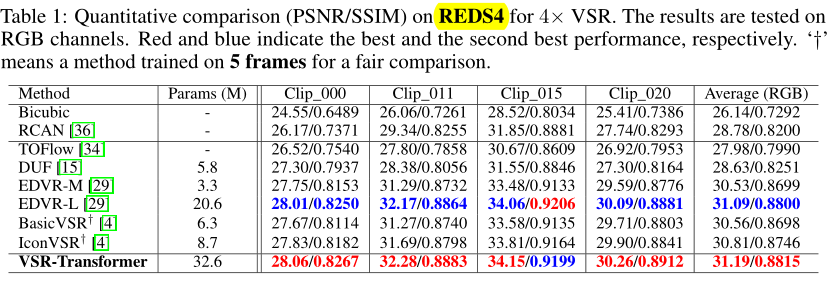### Vid4上的测试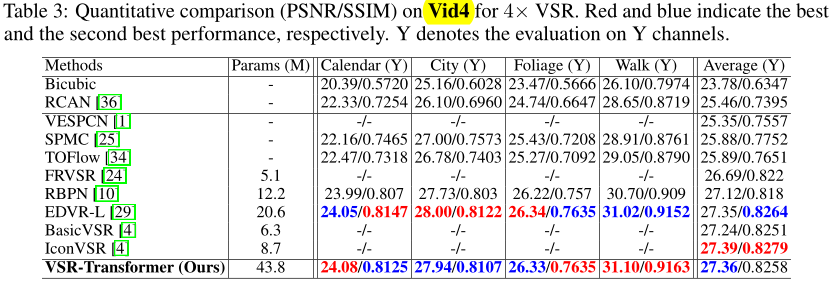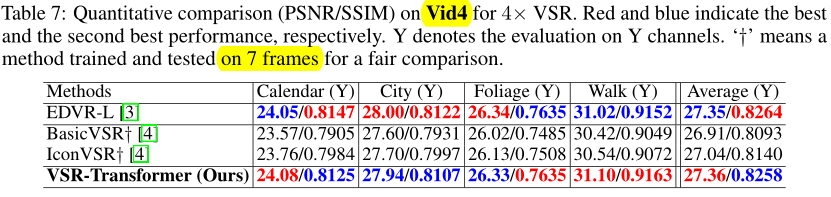### 消融实验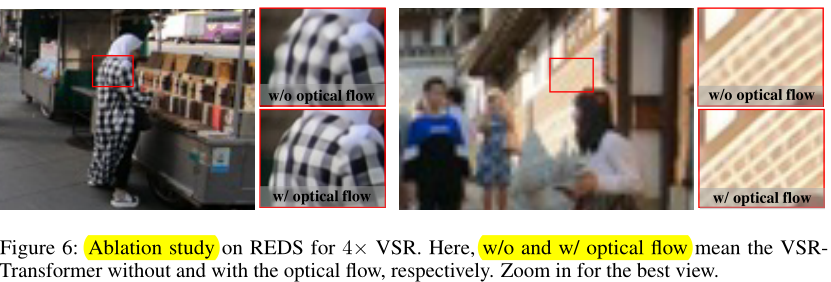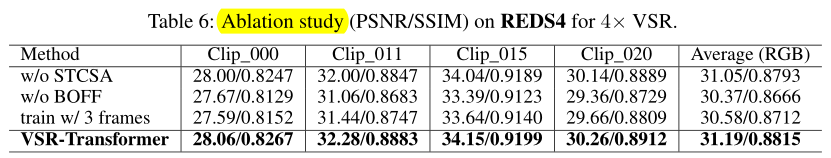### 低价透明### 金牌服务### 信息保密